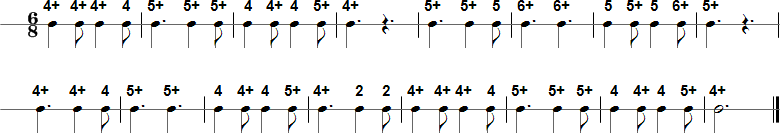Browse by Title:

# Itsy Bitsy Spider - Harmonica Tablature and Sheet Music## Tab in Text Format

4+ 4+ 4+ 4 5+ 5+ 5+ 4 4+ 4 5+ 4+ 5+ 5+ 5 6+ 6+ 5 5+ 5 6+ 5+ 4+ 4+ 4 5+ 5+ 4 4+ 4 5+ 4+ 2 2 4+ 4+ 4+ 4 5+ 5+ 5+ 4 4+ 4 5+ 4+